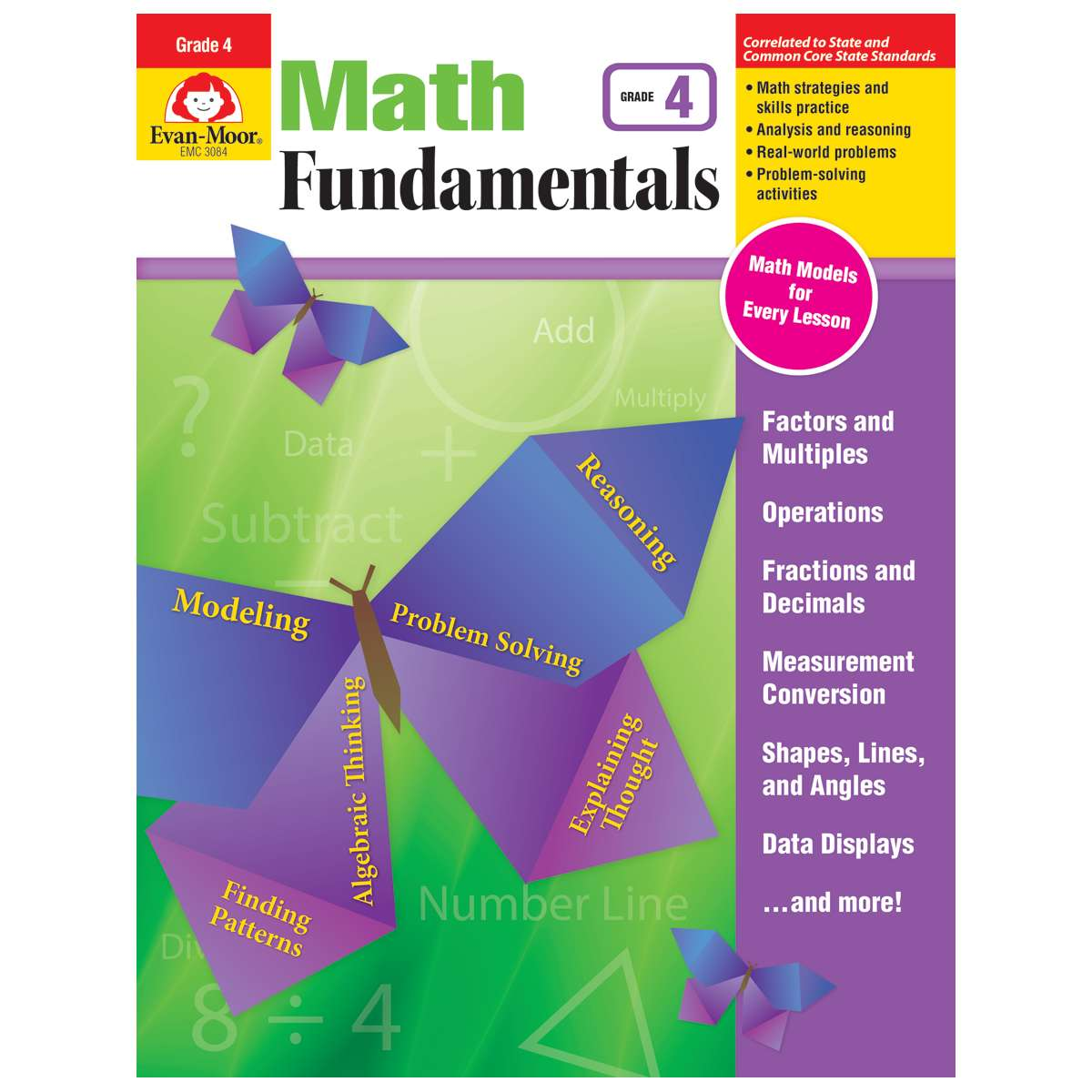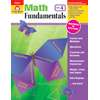•### Product Media

Product Number: EMC3084
Comprehensive but not complicated! Math Fundamentals helps your fourth graders navigate the new math. Math Models and analysi...
Quantity

Comprehensive but not complicated! Math Fundamentals helps your fourth graders navigate the new math. Math Models and analysis questions, plenty of skill practice, and real-world problems guide students in thinking through and solving problems.

To help you target instruction, each unit clearly lists the standards information, mathematical practices, and skills covered.

Within a unit, math lessons are presented simply. Every math lesson includes:
• A Math Models reference page that shows students strategies for solving problems. This is helpful for parents and students transitioning to new math.
• Skill practice pages that progress in difficulty.
• A culminating problem-solving task that leads students through the process of solving a real-life problem.
• Use the four operations to solve problems
• Find factors and multiples
• Generate and analyze patterns
• Use place value in number form and rounding
• Add, subtract, multiply, and divide multidigit numbers
• Make equivalent fractions and compare fractions
• Compare and order fractions
• Add, subtract, and multiply fractions
• Use decimal fractions and decimals
• Convert measurements within a system
• Represent and interpret data
• Measure angles
• Classify shapes
Designed to support new math programs, Math Fundamentals is the perfect easy-to-use supplement to your Common Core math or current math curriculum.

Better together! Use Math Fundamentals with Daily Math Practice for complementary skill practice that ensures your students master grade-level math.

This resource contains teacher support pages, reproducible student pages, and an answer key.
EMC3084